# Playing with I-V CurvesWritten by

## Plot-Powered Insights

### The I-V characteristics of an electronic component reveal a lot about its behavior. In this article, Robert steps through the basics of I-V curves—how to interpret, how to do your own I-V characteristic measurements and how to apply the results to your engineering work.

• What are the basics of I-V curves?

• How to interpret IV curves

• How to do your own I-V characteristic measurements

• How to apply the results to your engineering work

• Agilent (Keysight) DSO-X 3024A 200MHz digital oscilloscope

Welcome to “The Darker Side.” Since I began writing this column 15 years ago, I have regularly talked about techniques forgotten or misunderstood by a majority of electronics designers. Maybe it will also be the case this time. Do you know I-V curves? Do you use them from time to time, either to design a new product or to find a faulty component on a device? If not, then take a seat and enjoy, because I-V curves is this month’s topic.

##### I-V CURVES BASICS

Let’s start with some basics. I guess you all know Ohm’s law, right? V = I×R or I = V/R. This means that the current (I) going through a resistor is simply proportional to the voltage (V) applied across its two terminals. Now, imagine that you take this resistor and connect it to a variable voltage source. If you slowly increase the voltage while measuring the current going through the resistor, you will get a set of (I,V) coordinates. If you plot these coordinates on a graph, with V on the horizontal axis and I on the vertical axis, what would you get? A straight line, of course, because I and V are proportional. Such a graph is an “I-V curve”—a graphical representation of the relationship between the voltage applied across an electrical device and the current flowing through it.

Plotting the I-V curve of a resistor isn’t really fun, so I will move to the more interesting example of a silicon diode (Figure 1). If the voltage applied initially is 0V, then the current is, of course, 0A, since there isn’t any source of energy. If you then increase the voltage to 0.1V, the diode is forward biased, but the current stays very close to 0, because the voltage is lower than the threshold voltage of the diode. This means that the curve stays close to the horizontal axis.Figure 1 An I-V curve is simply the graphical representation of the current-to-voltage relationship of a device, in this case a diode.

Continue to increase the voltage. Something interesting happens when the voltage reaches about 0.6V, which is the P-N junction internal barrier voltage of a silicon diode: The current increases very quickly. On the graph, the line is now nearly parallel to the current axis, since the voltage stays close to 0.6V while the current increases quickly. To be precise, it increases exponentially, as given by the Shockley equation. This continues until the destruction of the diode, if the source is not current limited.

Such an I-V curve can be extended on the left side of the graph, meaning with negative voltages applied on the device under test. Here, with the example of a diode, a reversed voltage means that the diode is reverse biased, and no current will circulate. The plot will stay close to the horizontal axis as I=0. See Figure 1 for the complete, theoretical I-V plot of a diode.

##### TEST SETUP

Enough theory. Why not actually measure and plot the I-V curve of a device, starting with a diode? Of course, test equipment manufacturers developed dedicated instruments for this purpose. This started with the model 570 curve tracer from Tektronix introduced in 1955, now replaced by more versatile equipment called SMUs (source measurement units). However, I don’t suppose you have such a specialized test tool on your desk, right?

The good news is that it is actually simple to assemble a small I-V plotter, as long as you don’t need high performance. All you need is a dual-channel oscilloscope that’s able to work in so-called X-Y mode. In this mode—available on virtually any oscilloscope—the time-base and trigger sections of the instrument are not used. Rather, each of the two inputs drives respectively the vertical and horizontal position of the plot. This is exactly what is needed to plot I-V curves.

You then need a variable voltage source. The easiest way is to simply use the 50Hz or 60Hz alternating voltage coming from your power outlet. This AC voltage, after safety insulation and voltage reduction through a small transformer, provides a voltage varying continuously through a range of positive and negative voltages. The shape of the sweep is a sine, but this doesn’t matter as long as you simultaneously measure the voltage and current applied to the device, and plot them.

For the purposes of this article, I quickly assembled such a setup on my desk. The schematic is illustrated in Figure 2. I grabbed a small 230V/20V AC transformer and added a series 1kΩ resistor to provide current limiting. The resistor also provides a way to measure the current, simply by measuring the voltage across this resistor (remember Ohm’s law?). I then took the nearest of my oscilloscopes, and connected its two probes to the test set—channel 1 across the device under test (DUT) to measure the voltage, and channel 2 across the 1kΩ resistor to measure the current.

Here there is a problem. The two inputs of a standard oscilloscope aren’t insulated from one another. This means that their grounds are interconnected, so you can’t connect the two probes across two different devices, except if they share the same ground. One way to avoid this problem is to use so-called “differential probes,” but there is a simpler solution with a small trick.

Look again at Figure 2. To avoid this constraint, I assumed that the “ground” is the common point between the 1kΩ resistor and the DUT. This allows me to connect the ground clips of the two probes at the same point. However, there is a drawback: The measured voltage across the DUT has the wrong polarity. Fortunately, all oscilloscopes I know have an “invert input” button, which allows recovery of a plot in the good orientation. If you don’t have one, well, you will just have to look at the plot in a mirror!

Figure 3 shows you my actual setup. The oscilloscope I used is a nice Agilent DSO-X 3024A 200MHz digital oscilloscope, but even a vintage 10MHz analog oscilloscope could be used here. (Agilent Technologies’ electronic instrumentation group was split out as its own company Keysight Technologies in 2014.) The box on the left is a safety insulation transformer, since I never connect something to the 230V line on my desk without one. Life is too short to risk making it even shorter, so I advise you to do the same.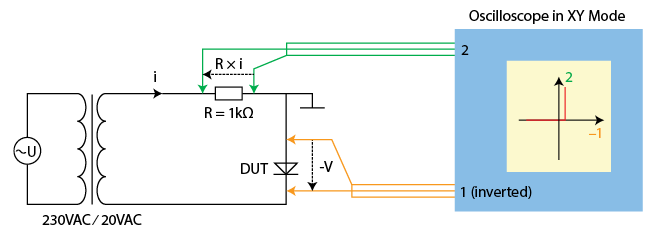Figure 2 Schematic of a simple-to-build I-V plotter setup
##### SWITCH ON

Now it is time to connect a diode as device under test (DUT). I took an old 1N4001 silicon rectifier diode, and switched on the power source. With the oscilloscope in normal mode (time-sweeping, not X-Y), you will get curves close to the ones depicted in Figure 4. The top yellow curve is the voltage across the diode, while the bottom green curve is the current.

How to interpret these waveforms? When the input voltage is negative, the voltage across the diode follows the input voltage and is depicted as a half-wave negative shape. Simultaneously, the current stays at zero. Conversely, when the input voltage is positive, then the voltage across the diode is close to zero (0.6V, in fact, but this isn’t clearly visible on the plot), while the current increases and follows a positive half-wave shape.

Now I pressed on the “X-Y mode” button. Look at the result in Figure 5. Close to the theory, isn’t it? At negative voltages, the current remains nearly at zero. However, when the diode is forward biased and exceeds about 0.6V, avalanche occurs, and the forward current increases rapidly for a very small increase in voltage. The resulting shape is an L, typical for a p-n semiconductor junction.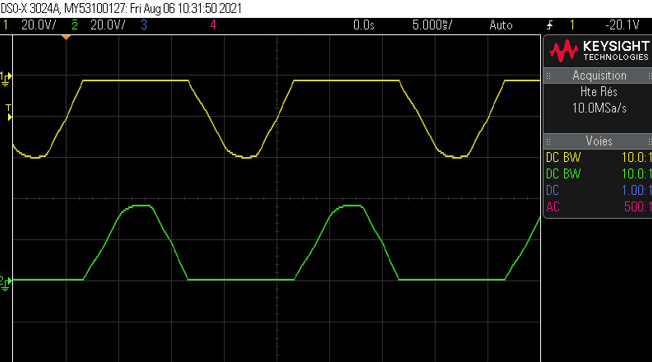Figure 4 The I-V curves of a diode, with the oscilloscope still in normal mode (not X-Y)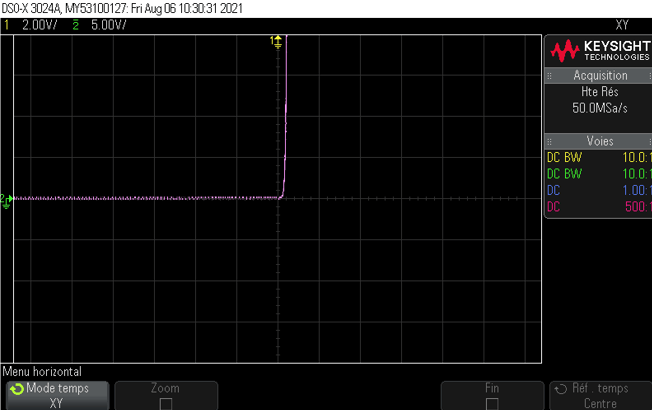Figure 5 When switched to X-Y mode, the I-V curve of the diode is clearly visible.
##### LEARNING FROM I-V CURVES

As illustrated with this simple example of a diode, an I-V curve is a good way of understanding how a device behaves. Plenty of its key properties can be extracted from the shape of its I-V curve. For example, all devices without a built-in power source have I-V curves that pass through the origin (current is null if applied voltage is null). Moreover, such devices are limited to the top right and bottom left quadrants of the I–V plane. This is simple to deduce, because the voltage and current always have the same polarity. So, if you have any device for which the I-V plot doesn’t stay in their quadrants, then you will know that the device is producing power, like a battery or solar cell.

The shape of the curve is also informative: As explained, a straight line through the origin represents a resistive element, whereas a curved line represents a nonlinear device such as a semiconductor. More interestingly, a non-monotonic I–V curve (one that has several peaks and valleys) is quite rare, and is a characteristic of a device that shows negative resistance. Examples are tunnel and Gunn diodes.

Finally, some devices have hysteresis, which means that the current-to-voltage relationship depends not only on the present applied input but also on the past values. On the I–V curves, such devices show closed loops. Examples include iron-core inductors, neon lights, or semiconductors such as thyristors and DIACs (DIAC stands for “diode for alternating current”).

To illustrate different possible plots, I replaced the 1N4001 diode with a yellow LED (Figure 6). The shape of the curve is still an L, but the threshold voltage is significantly higher. Here I measured it at about 2.12V, in line with the datasheet of the LED.

Another interesting example is a Zener diode. This type of diode behaves as a standard diode when forward biased, but has a defined breakdown voltage when reverse biased. At this point, there is a sudden increase in reverse current, producing a nearly straight line again. While all diodes have a breakdown region, Zener diodes are designed to operate in this mode. See Figure 7 for a test of a 16V Zener diode: The shape of the curve is no longer an L, but rather an S. The top right quadrant shows the forward voltage of 0.6V, while the left bottom quadrant shows the backward breakdown voltage of about 16V.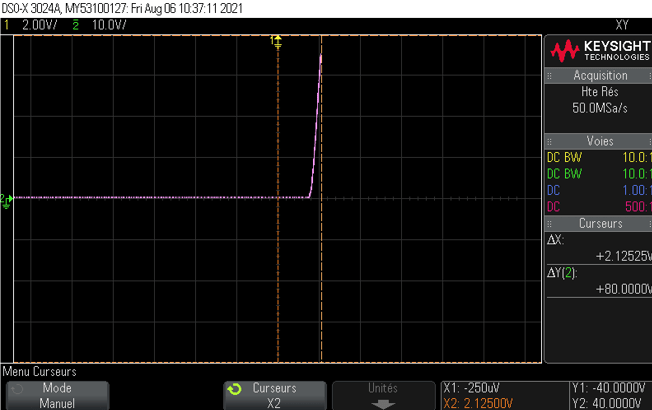Figure 6 The I-V curve of an LED is close to a standard diode, but with an increased drop-off voltage, here 2.12V.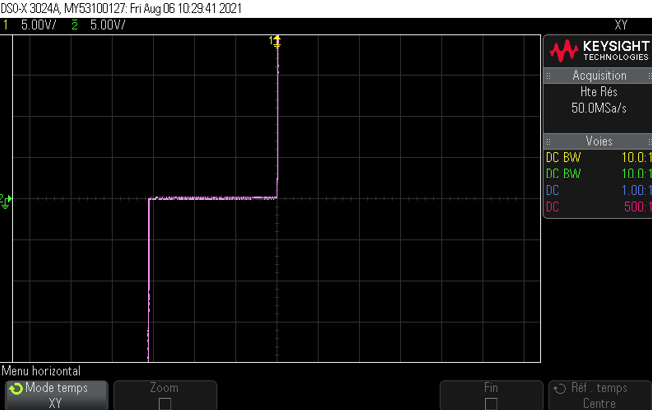Figure 7 A 16V Zener diode, with a clearly visible breakdown voltage close to 16V in the bottom left quadrant
##### TESTING A BIPOLAR TRANSISTOR

Testing a on an I-V plotter is also an interesting experiment. As you know, transistors are three-terminal semiconductor devices, where one terminal (the base) controls the flow of current between the remaining two terminals (collector and emitter). Here, the current-to-voltage relationship between one pair of terminals depends on the current or voltage applied on the third terminal. Such a transistor can be tested and plotted on an I-V curve, but the resulting graph will show multiple curves, each one representing the I-V characteristic for a given value of current or voltage applied on the third terminal.

It is actually easy to plot I-V curves of transistors using the simple test setup I previously described, with the addition of an auxiliary DC voltage source, which drives the third terminal. As an example, Figure 8 shows the test setup to measure the I-V characteristics of the collector-to-emitter junction, with varying current applied on the base of an npn transistor. You have then just to manually sweep the DC voltage source to get a plot of the transistor behavior for each base current. Moreover, if your oscilloscope is able to memorize and superpose several measurements, then you will directly get a nice plot like the one shown in Figure 9. Such a plot in particular allows the design of an amplifier using the tested transistor.

I am close to the end of this month’s article. So, unfortunately, I can’t really go into the details. Anyway, in a nutshell and as illustrated in Figure 10, each base current corresponds to a given saturated collector current (calculated as the voltage gain times the base current). If the collector of the transistor is connected to a power source VCC through a resistor RC, then the voltage on the collector will be defined by a straight line (in red in Figure 10). And the intersection of this line and the I-V curves of the transistor will allow you to find a good value for the operating point, meaning a good value for the average base current.Figure 8 The test setup can be easily modified to test a transistor.Figure 9 The superposed I-V curves of a bipolar transistor with varying base currentFigure 10 The I-V curve of a transistor allows an amplifier to be designed by setting a proper value for its operating point.
##### WRAPPING UP

Here we are. I hope you now understand that the I-V characteristics of an electronic component tells you a lot about its behavior. Just download the datasheet of any semiconductor device, and I am sure you will find some I-V curves. Understanding their meaning is a must for any designer. Moreover, knowing how to measure the actual I-V characteristic of a device is invaluable, especially when trying to repair or reverse engineer a product.

As usual, I strongly suggest that you perform your own experiments. Grab an oscilloscope, and play with I-V curves now. And if you don’t have an oscilloscope, then buy one. Even a 30-year-old basic model will be a great learning tool on your desk, and will probably not jeopardize your monthly budget. Just take care to get at least a dual-channel model with the great X-Y mode button!

To finish, a note to my dear regular readers: If I have counted correctly, my next article will be the 99th article I have published in Circuit Cellar! Two consequences: first, we are close to a celebration. And second, it will be time for some changes. Stay tuned!Editor’s note: For a project article about building your own I-V curve tracer system, check out “Create Your Own I-V Curve Tracer” by George Steber, Circuit Cellar 353, December 2019 at
www.circuitcellar.com/research-design-hub/create-your-own-i-v-curve-tracer

RESOURCES

https://en.wikipedia.org/wiki/Current–voltage_characteristic

https://www.electronics-tutorials.ws/blog/i-v-characteristic-curves.html

https://vintagetek.org/curve-tracers

Keysight Technologies | www.keysight.com

PUBLISHED IN CIRCUIT CELLAR MAGAZINE • DECEMBER 2021 #377 – Get a PDF of the issue

 Keep up-to-date with our FREE Weekly Newsletter! Don't miss out on upcoming issues of Circuit Cellar. Subscribe to Circuit Cellar Magazine Note: We’ve made the May 2020 issue of Circuit Cellar available as a free sample issue. In it, you’ll find a rich variety of the kinds of articles and information that exemplify a typical issue of the current magazine. Would you like to write for Circuit Cellar? We are always accepting articles/posts from the technical community. Get in touch with us and let's discuss your ideas.